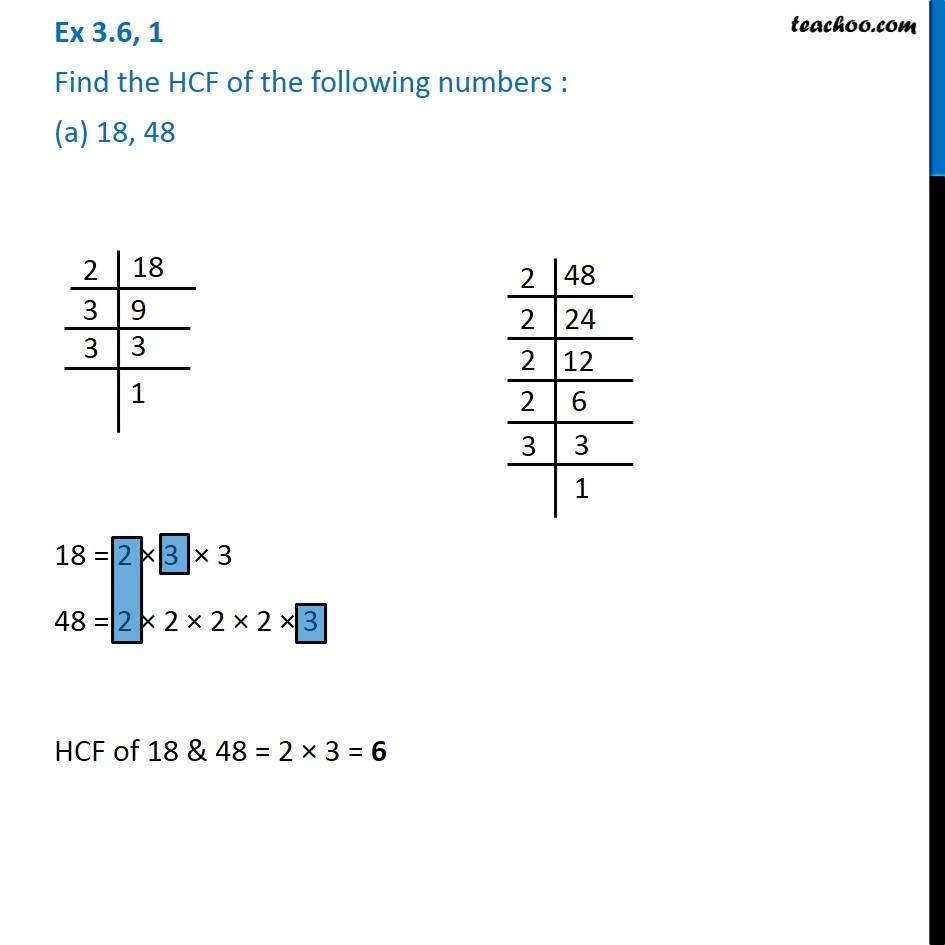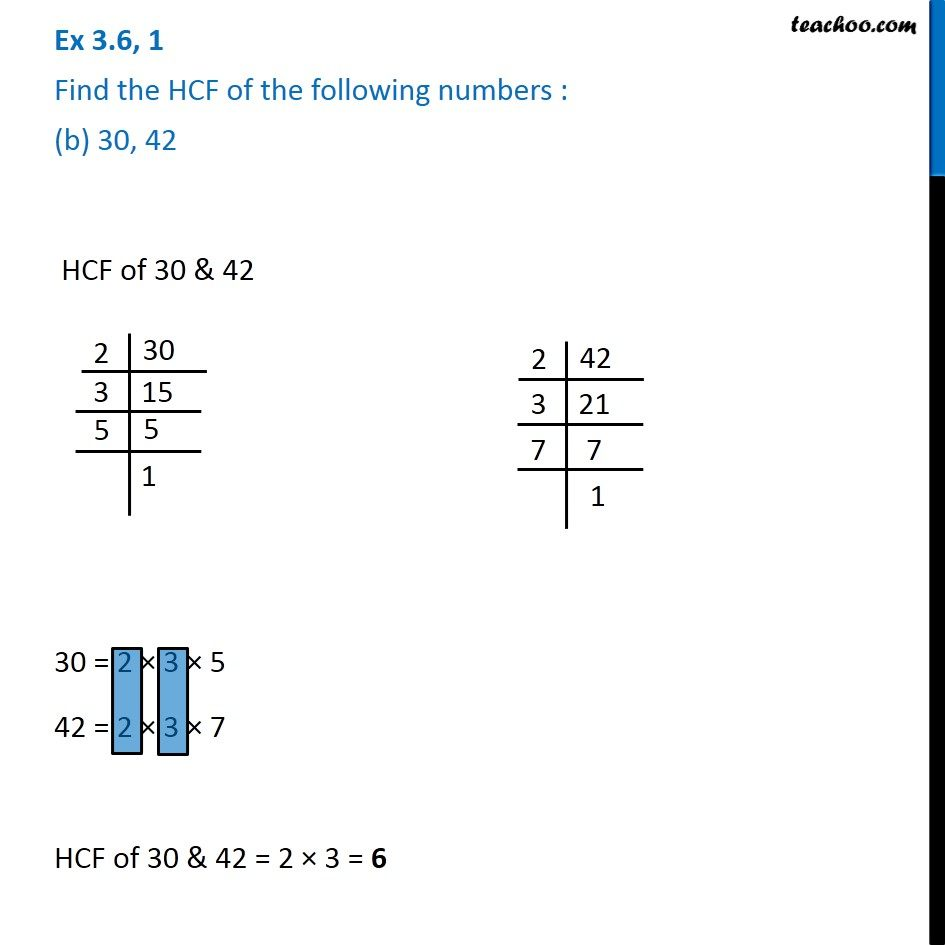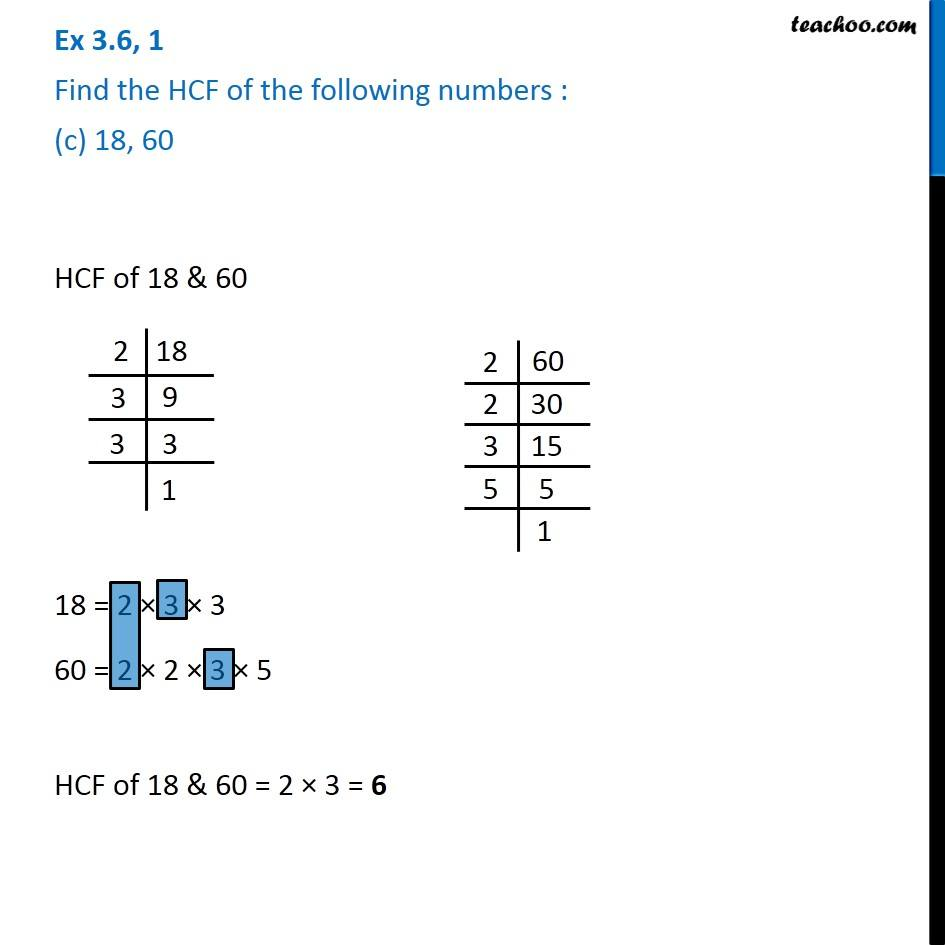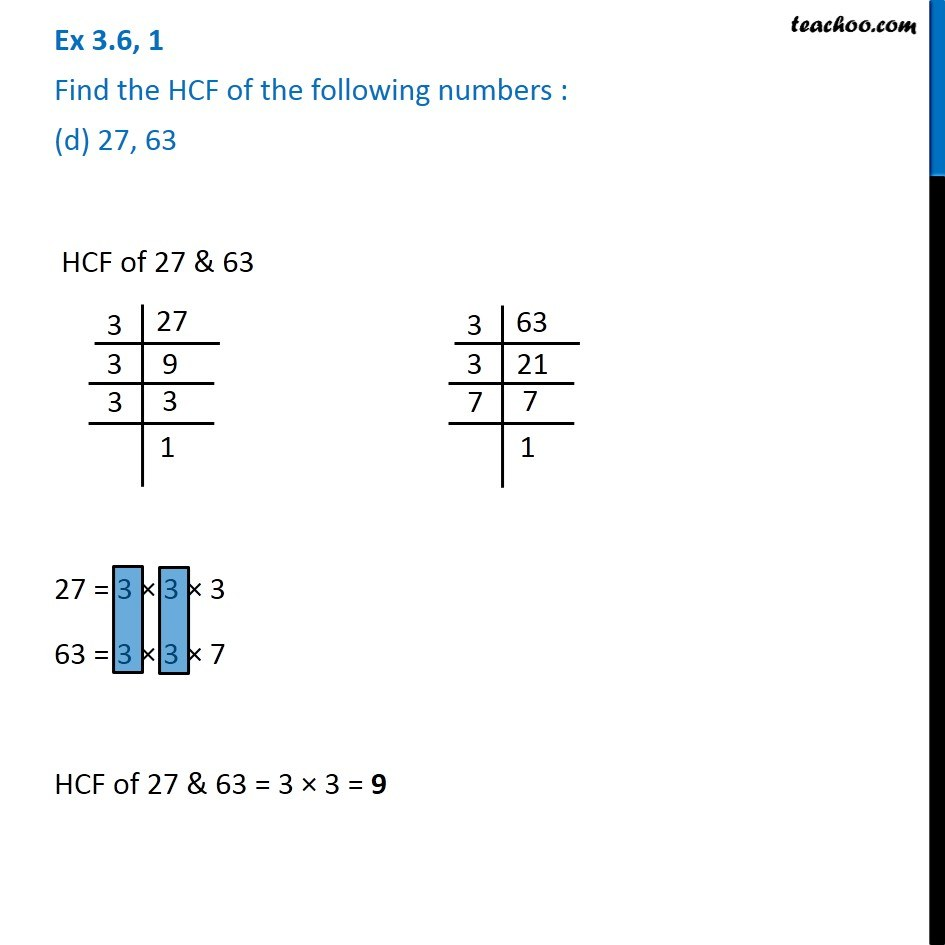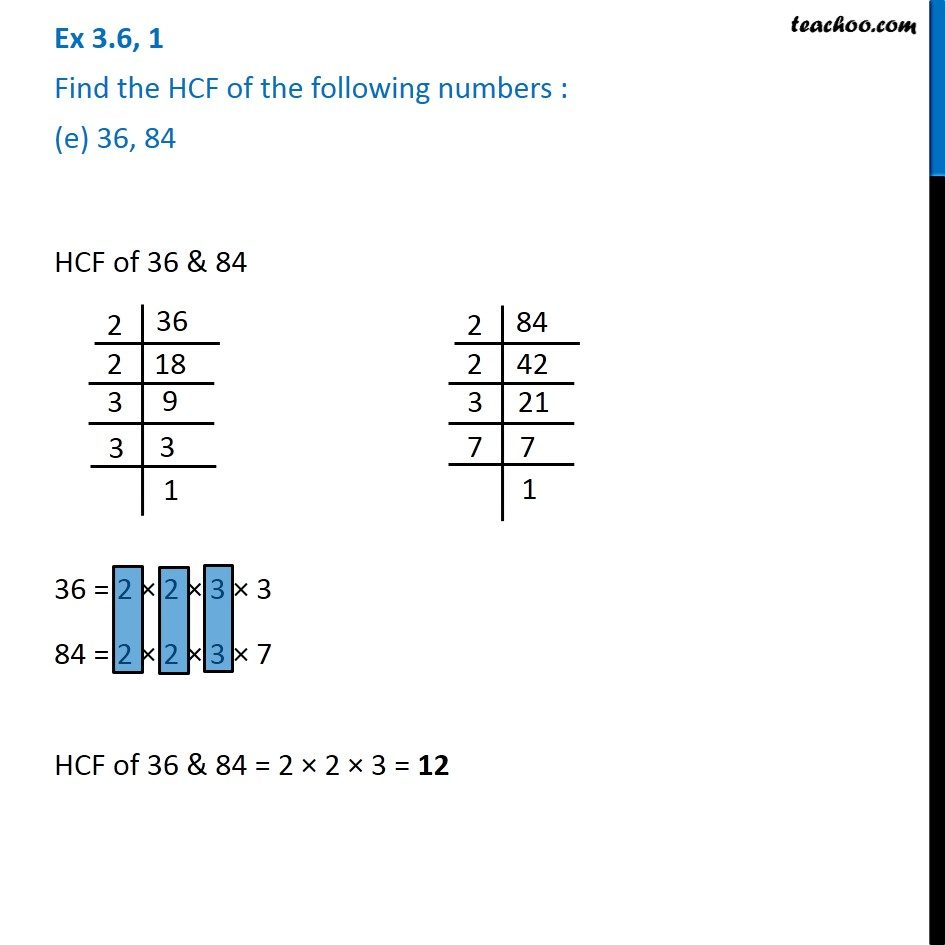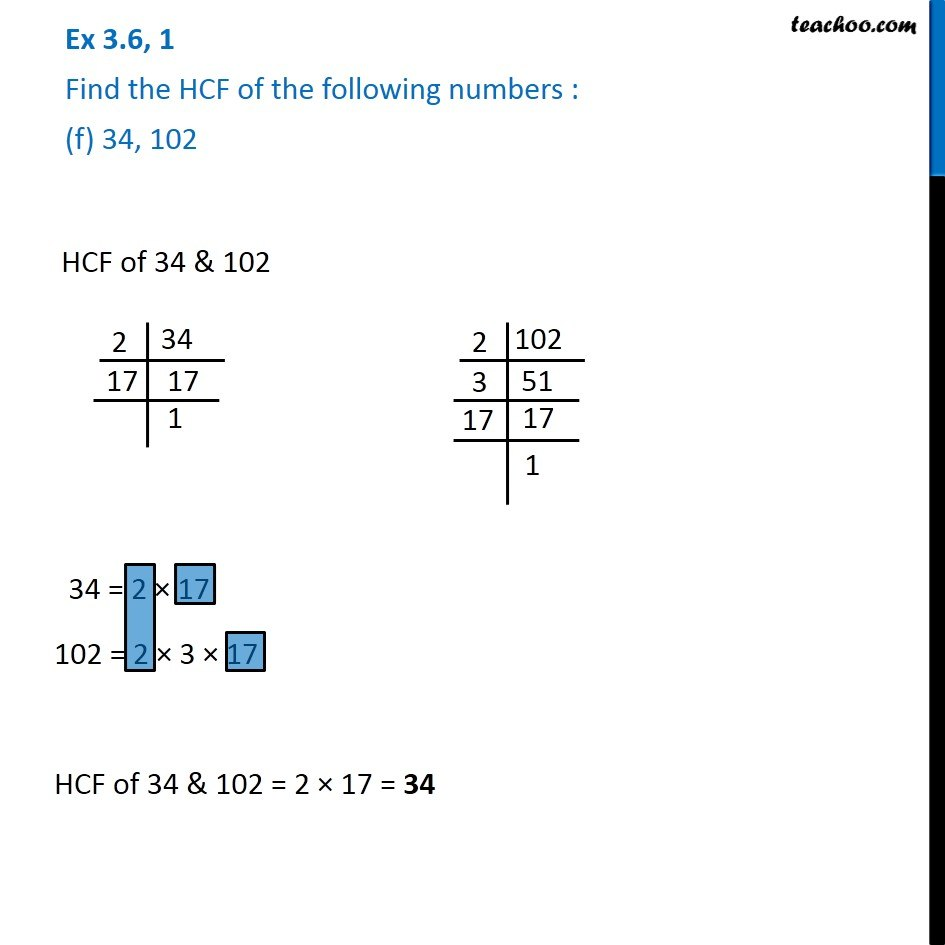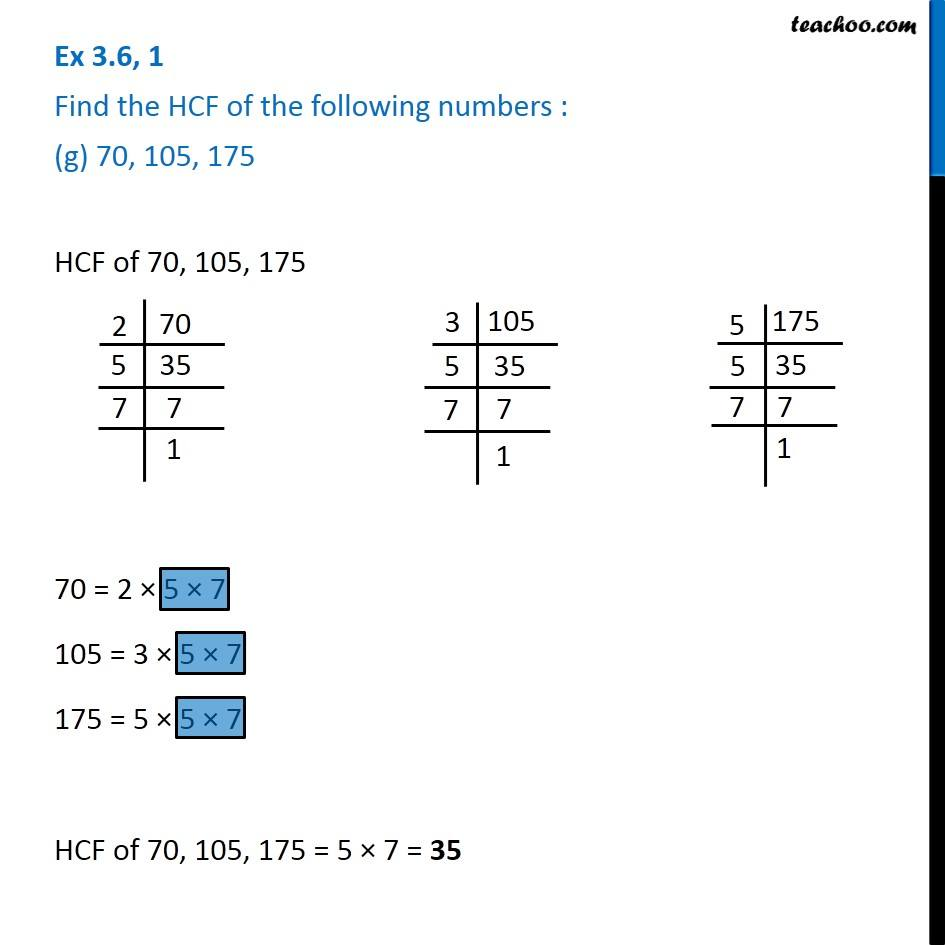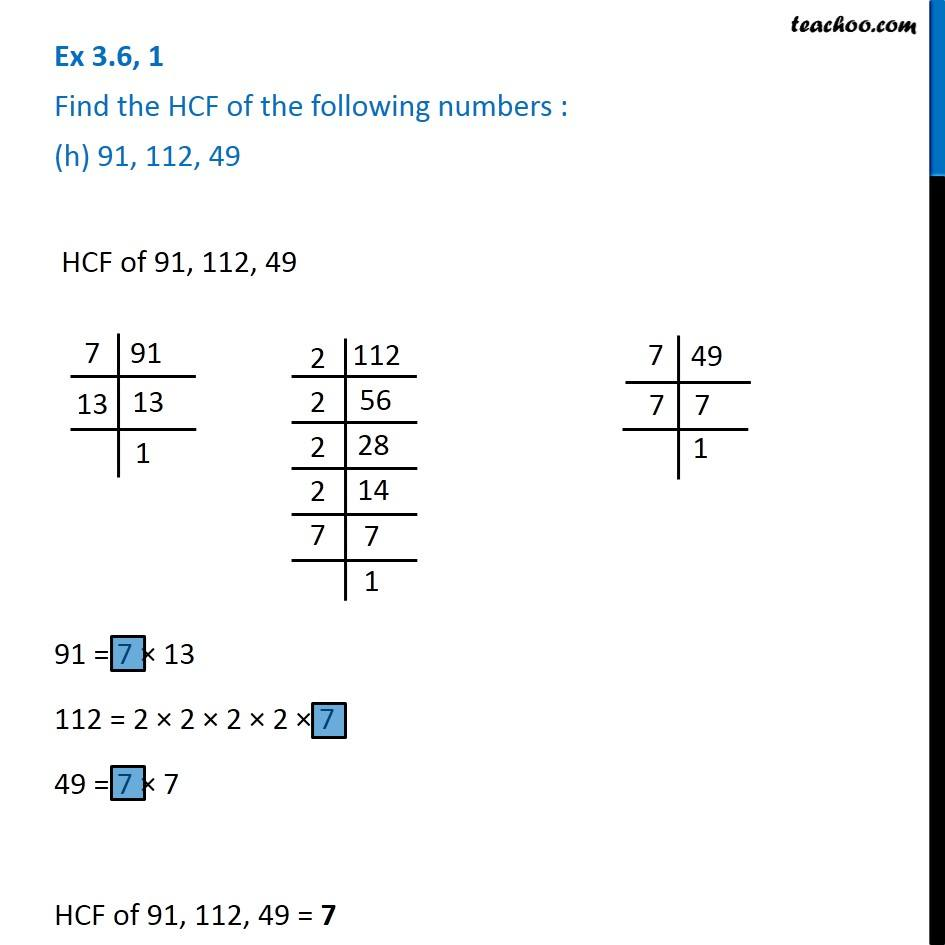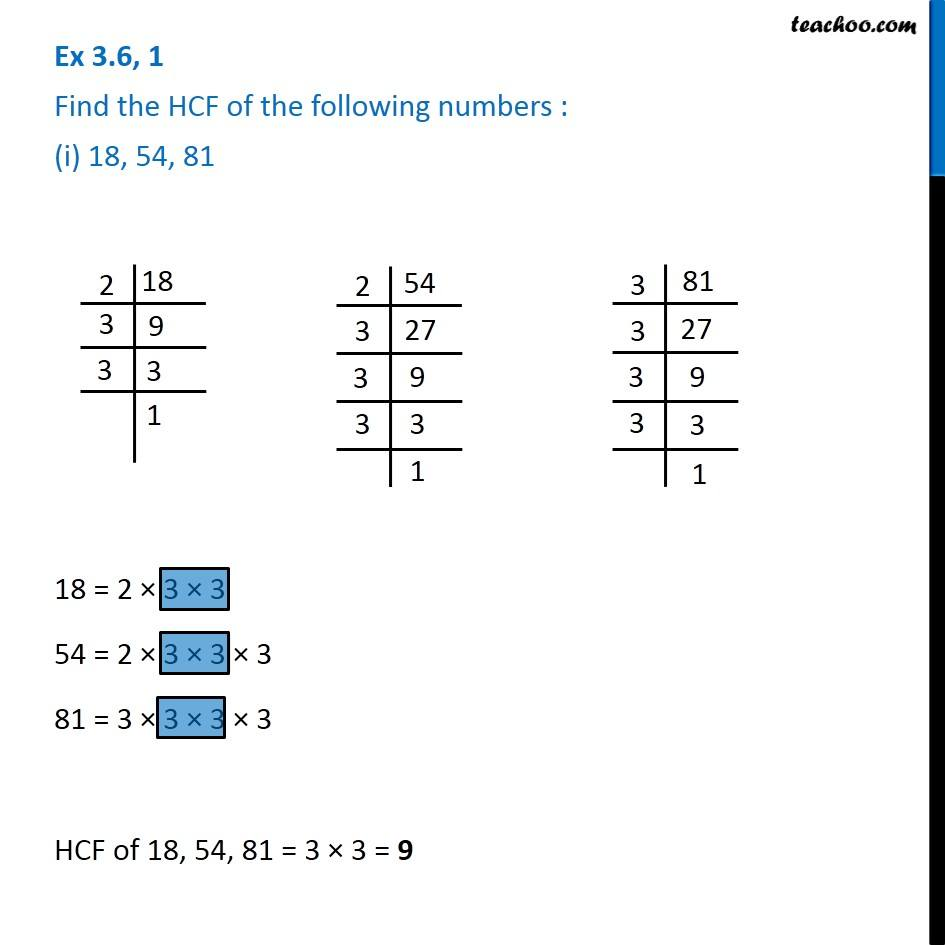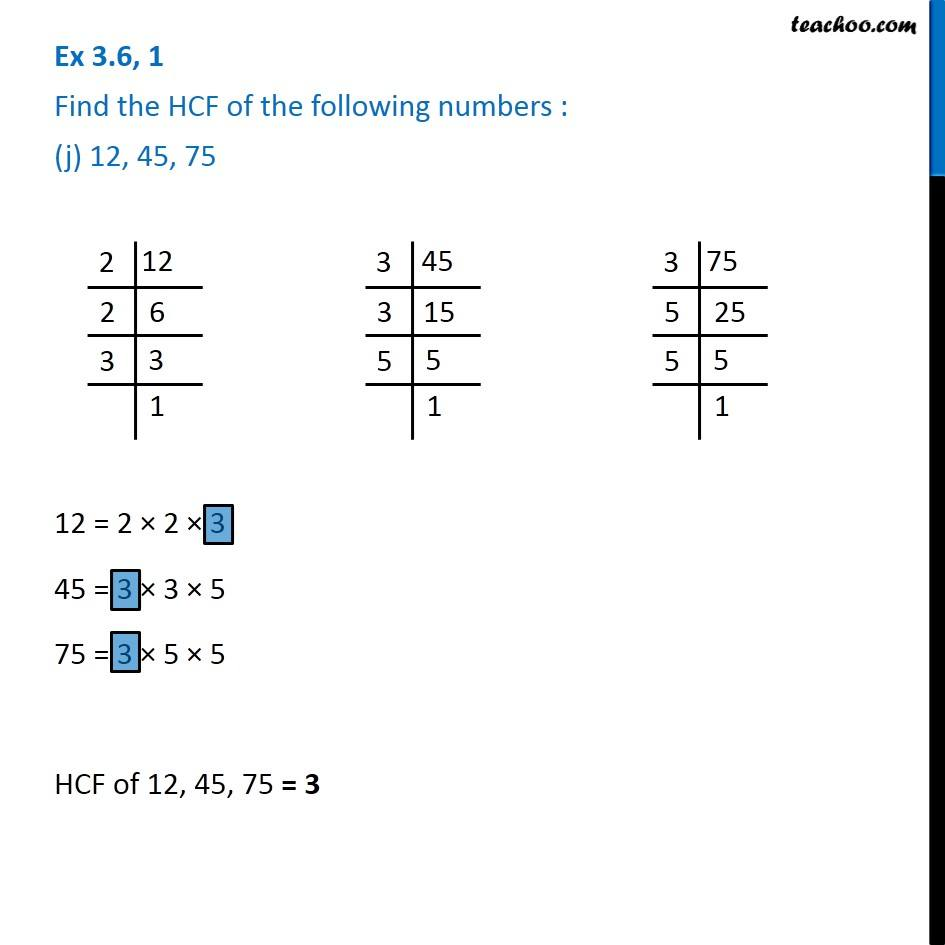Subscribe to our Youtube Channel - https://you.tube/teachoo

1. Chapter 3 Class 6 Playing with Numbers
2. Serial order wise
3. Ex 3.6

Transcript

Ex 3.6, 1 Find the HCF of the following numbers : (a) 18, 48 18 = 2 × 3 × 3 48 = 2 × 2 × 2 × 2 × 3 HCF of 18 & 48 = 2 × 3 = 6 Ex 3.6, 1 Find the HCF of the following numbers : (b) 30, 42 Ex 3.6, 1 Find the HCF of the following numbers : (b) 30, 42 Ex 3.6, 1 Find the HCF of the following numbers : (c) 18, 60 HCF of 18 & 60 18 = 2 × 3 × 3 60 = 2 × 2 × 3 × 5 HCF of 18 & 60 = 2 × 3 = 6 Ex 3.6, 1 Find the HCF of the following numbers : (d) 27, 63 HCF of 27 & 63 27 = 3 × 3 × 3 63 = 3 × 3 × 7 HCF of 27 & 63 = 3 × 3 = 9 Ex 3.6, 1 Find the HCF of the following numbers : (e) 36, 84 HCF of 36 & 84 36 = 2 × 2 × 3 × 3 84 = 2 × 2 × 3 × 7 HCF of 36 & 84 = 2 × 2 × 3 = 12 Ex 3.6, 1 Find the HCF of the following numbers : (f) 34, 102 HCF of 34 & 102 34 = 2 × 17 102 = 2 × 3 × 17 HCF of 34 & 102 = 2 × 17 = 34 Ex 3.6, 1 Find the HCF of the following numbers : (g) 70, 105, 175 HCF of 70, 105, 175 70 = 2 × 5 × 7 105 = 3 × 5 × 7 175 = 5 × 5 × 7 HCF of 70, 105, 175 = 5 × 7 = 35 Ex 3.6, 1 Find the HCF of the following numbers : (h) 91, 112, 49 HCF of 91, 112, 49 91 = 7 × 13 112 = 2 × 2 × 2 × 2 × 7 49 = 7 × 7 HCF of 91, 112, 49 = 7 Ex 3.6, 1 Find the HCF of the following numbers : (i) 18, 54, 81 18 = 2 × 3 × 3 54 = 2 × 3 × 3 × 3 81 = 3 × 3 × 3 × 3 HCF of 18, 54, 81 = 3 × 3 = 9 Ex 3.6, 1 Find the HCF of the following numbers : (j) 12, 45, 75 12 = 2 × 2 × 3 45 = 3 × 3 × 5 75 = 3 × 5 × 5 HCF of 12, 45, 75 = 3

Ex 3.6